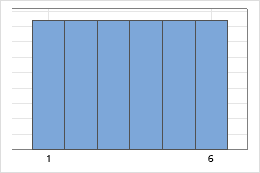# Integer distribution

Use the integer distribution to model equally likely integers. For example, if a fair die is thrown, the probability of obtaining any of the six possible outcomes is the same: 1/6.

The integer distribution is a discrete uniform distribution that ranges from the minimum to the maximum integer value specified. Each integer in the range has equal probability. For example, the following graph illustrates that the probability of a fair die is the same for each number.The integer distribution does not occur often in nature, but it is important as a reference distribution. One of the most important applications is to generate random integers that fall between two specific values.

By using this site you agree to the use of cookies for analytics and personalized content.  Read our policy